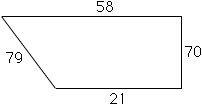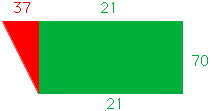Subject: Finding Sq Ft Can you tell me what the sq. ft. are of and area measuring: ```__________58____________ | | | | | | | | | | 76 | 70 | | | | | |______21______ | ``` Tx, Frank Frank W. Ploof Hi Frank, From the diagram that you sent I am assuming that the sides of length 58 feet and 21 feet are parallel and the side of length 70 ft is perpendicular to each of them. If this is so then the fourth side is closer to 79 feet than 76 feet. (the diagram below is not to scale.)I think that the easiest way to find the area is to imagine that you cut the region into two pieces, a rectangle and a triangle.The rectangle has area 21 x 70 = 1470 square feet and the triangle has area 37 x 70/2 = 1295 square feet. Thus the total area is 1470 + 1295 = 2765 square feet. If my assumption at the beginning is incorrect let me know. Harley Go to Math Central